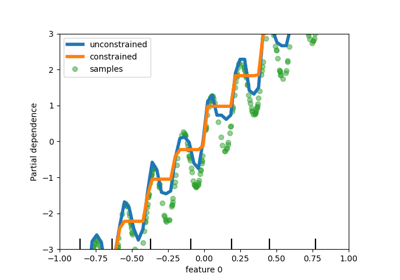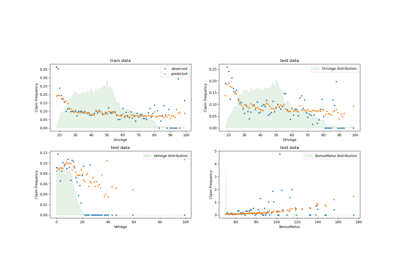# sklearn.linear_model.GammaRegressor¶

class sklearn.linear_model.GammaRegressor(*, alpha=1.0, fit_intercept=True, max_iter=100, tol=0.0001, warm_start=False, verbose=0)[source]

Generalized Linear Model with a Gamma distribution.

Read more in the User Guide.

Parameters
alphafloat, default=1

Constant that multiplies the penalty term and thus determines the regularization strength. alpha = 0 is equivalent to unpenalized GLMs. In this case, the design matrix X must have full column rank (no collinearities).

fit_interceptbool, default=True

Specifies if a constant (a.k.a. bias or intercept) should be added to the linear predictor (X @ coef + intercept).

max_iterint, default=100

The maximal number of iterations for the solver.

tolfloat, default=1e-4

Stopping criterion. For the lbfgs solver, the iteration will stop when max{|g_j|, j = 1, ..., d} <= tol where g_j is the j-th component of the gradient (derivative) of the objective function.

warm_startbool, default=False

If set to True, reuse the solution of the previous call to fit as initialization for coef_ and intercept_ .

verboseint, default=0

For the lbfgs solver set verbose to any positive number for verbosity.

Attributes
coef_array of shape (n_features,)

Estimated coefficients for the linear predictor (X * coef_ + intercept_) in the GLM.

intercept_float

Intercept (a.k.a. bias) added to linear predictor.

n_iter_int

Actual number of iterations used in the solver.

Methods

 fit(X, y[, sample_weight]) Fit a Generalized Linear Model. get_params([deep]) Get parameters for this estimator. Predict using GLM with feature matrix X. score(X, y[, sample_weight]) Compute D^2, the percentage of deviance explained. set_params(**params) Set the parameters of this estimator.
__init__(*, alpha=1.0, fit_intercept=True, max_iter=100, tol=0.0001, warm_start=False, verbose=0)[source]

Initialize self. See help(type(self)) for accurate signature.

fit(X, y, sample_weight=None)[source]

Fit a Generalized Linear Model.

Parameters
X{array-like, sparse matrix} of shape (n_samples, n_features)

Training data.

yarray-like of shape (n_samples,)

Target values.

sample_weightarray-like of shape (n_samples,), default=None

Sample weights.

Returns
selfreturns an instance of self.
get_params(deep=True)[source]

Get parameters for this estimator.

Parameters
deepbool, default=True

If True, will return the parameters for this estimator and contained subobjects that are estimators.

Returns
paramsmapping of string to any

Parameter names mapped to their values.

predict(X)[source]

Predict using GLM with feature matrix X.

Parameters
X{array-like, sparse matrix} of shape (n_samples, n_features)

Samples.

Returns
y_predarray of shape (n_samples,)

Returns predicted values.

score(X, y, sample_weight=None)[source]

Compute D^2, the percentage of deviance explained.

D^2 is a generalization of the coefficient of determination R^2. R^2 uses squared error and D^2 deviance. Note that those two are equal for family='normal'.

D^2 is defined as $$D^2 = 1-\frac{D(y_{true},y_{pred})}{D_{null}}$$, $$D_{null}$$ is the null deviance, i.e. the deviance of a model with intercept alone, which corresponds to $$y_{pred} = \bar{y}$$. The mean $$\bar{y}$$ is averaged by sample_weight. Best possible score is 1.0 and it can be negative (because the model can be arbitrarily worse).

Parameters
X{array-like, sparse matrix} of shape (n_samples, n_features)

Test samples.

yarray-like of shape (n_samples,)

True values of target.

sample_weightarray-like of shape (n_samples,), default=None

Sample weights.

Returns
scorefloat

D^2 of self.predict(X) w.r.t. y.

set_params(**params)[source]

Set the parameters of this estimator.

The method works on simple estimators as well as on nested objects (such as pipelines). The latter have parameters of the form <component>__<parameter> so that it’s possible to update each component of a nested object.

Parameters
**paramsdict

Estimator parameters.

Returns
selfobject

Estimator instance.

## Examples using sklearn.linear_model.GammaRegressor¶